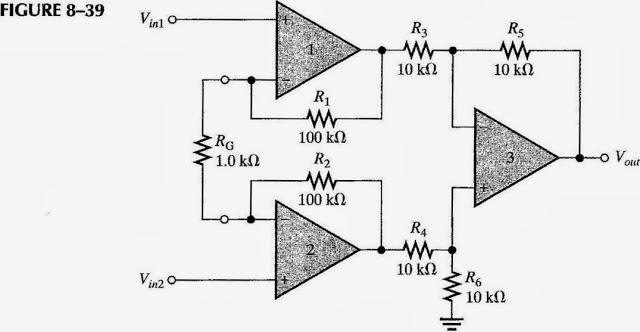# Determining voltage gain of instrumentation amplifier

In summary, the problem involves an instrumentation amplifier with Vin1=5 mV, Vin2=10 mV, and Vcm=225 mV. The equations to solve for the final output voltage are R_{1}=R_{2}=R, A_{cl}=1+\frac{2R}{R_{G}}, and V_{out}=\left ( 1+\frac{2R}{R_{G}} \right )\left ( V_{in2}-V_{in1} \right ). With incorrect initial calculations, the output voltage was found to be 1 mV, but this does not take into account Vcm. After correcting the math error, the final output voltage is determined to be 1

Gold Member

## Homework Statement

The following voltages are applied to the instrumentation amplifier in the figure.
Vin1=5 mV, Vin2=10 mV, and Vcm=225 mV. Determine the final output voltage.## Homework Equations

$$R_{1}=R_{2}=R$$

$$A_{cl}=1+\frac{2R}{R_{G}}$$

$$V_{out}=\left ( 1+\frac{2R}{R_{G}} \right )\left ( V_{in2}-V_{in1} \right )$$

## The Attempt at a Solution

This is an odd-numbered problem in the back of my book, so I know the answer should be 1.005 V.

I already know my initial answer (see below) is wrong because it comes out to 1 V and does not take into account Vcm. Since I'm given Vcm but not Acm, CMRR, or anything else, how do I solve this problem?

Incorrect:
$$V_{out}=\left ( 1+\frac{2\cdot 100k\Omega }{1.0k\Omega} \right )\left ( 10mV-5mV \right )=1mV$$

What does Vcm=225 mV represent?

I believe it represents a common-mode voltage (a noise signal or some other common signal on both inputs).

$$V_{out}=\left ( 1+\frac{2\cdot 100k\Omega }{1.0k\Omega} \right )\left ( 10mV-5mV \right )= ?$$